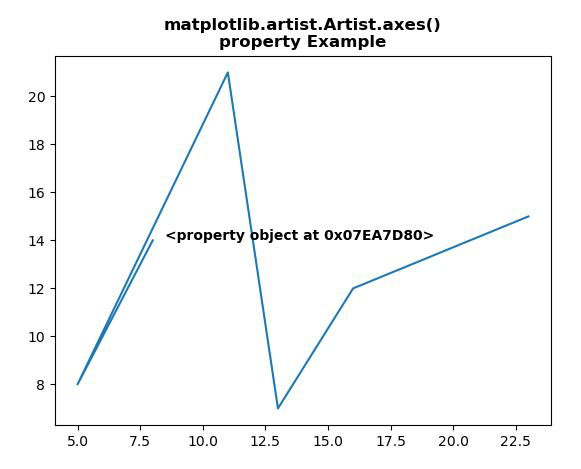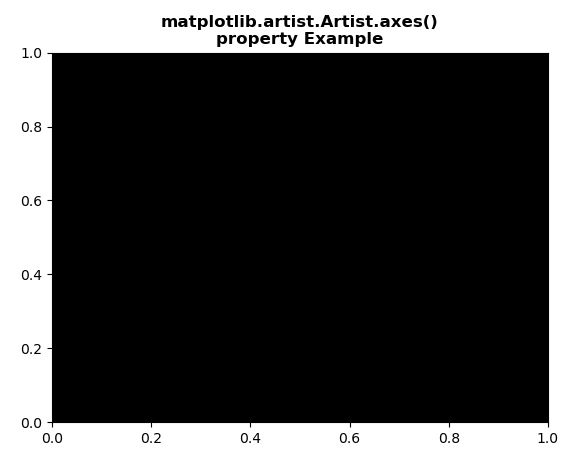# Matplotlib.artist.Artist.axes in Python

• Last Updated : 15 May, 2020

Matplotlib is a library in Python and it is numerical – mathematical extension for NumPy library. The Artist class contains Abstract base class for objects that render into a FigureCanvas. All visible elements in a figure are subclasses of Artist.

## Matplotlib.artist.Artist.axes() property

The axes() property in artist module of matplotlib library is the Axes instance the artist resides in, or None

Attention geek! Strengthen your foundations with the Python Programming Foundation Course and learn the basics.

To begin with, your interview preparations Enhance your Data Structures concepts with the Python DS Course. And to begin with your Machine Learning Journey, join the Machine Learning - Basic Level Course

property: Artist.axes

Below examples illustrate the matplotlib.artist.Artist.axes() property in matplotlib:

Example 1:

 `# Implementation of matplotlib function``from` `matplotlib.artist ``import` `Artist ``import` `matplotlib.pyplot as plt  `` ` ` ` `# providing values to x and y  ``x ``=` `[``8``, ``5``, ``11``, ``13``, ``16``, ``23``] ``y ``=` `[``14``, ``8``, ``21``, ``7``, ``12``, ``15``] ``   ` `# to plot x and y ``plt.plot(x, y) `` ` `# use of axes() property``axs ``=` `Artist.axes``plt.text(``8.5``, ``14``, ``str``(axs), fontweight``=``"bold"``)`` ` `plt.title(``"""matplotlib.artist.Artist.axes()``property Example"""``, fontweight``=``"bold")`` ` `plt.show()`

Output:Example 2:

 `# Implementation of matplotlib function``from` `matplotlib.artist ``import` `Artist ``import` `matplotlib.pyplot as plt  `` ` ` ` `# providing values to x and y  ``x ``=` `[``8``, ``5``, ``11``, ``13``, ``16``, ``23``] ``y ``=` `[``14``, ``8``, ``21``, ``7``, ``12``, ``15``] ``   ` `# to plot x and y ``plt.plot(x, y) ``plt.axes(facecolor ``=` `'black'``)`` ` `axs ``=` `Artist.axes``print``(``str``(axs))`` ` `plt.title(``"""matplotlib.artist.Artist.axes()``property Example"""``, fontweight``=``"bold")`` ` `plt.show()`

Output:My Personal Notes arrow_drop_up